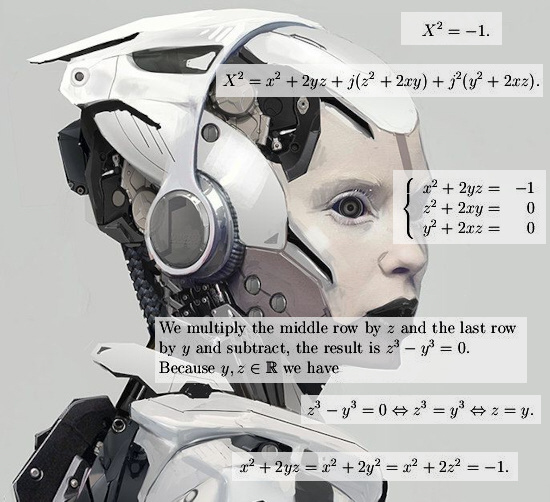# Proof that Z^2 = -1 cannot be solved on real spaces with an odd dimension. (General theory part 1.)

Finally after all those years something of a more general approach to multiplication in higher dimensions? Yes but at the same time I remark you should not learn or study higher dimensional numbers that way. You better pick a particular space like 3D complex numbers and find a lot out about them and then move on to say 4D or 5D complex numbers and repeat that process.
Problem with a more general approach is that those spaces are just too different from each other so it is hard to find some stuff all of those spaces have. It is like making theory for the complex plane and the split complex numbers at the same time: It is not a good idea because they behave very differently.
The math in this post is utterly simple, basically I use only that the square of a real number, this time a determinant, cannot be negative. The most complicated thing I use of the rule that says the determinant of a square is the square of the determinant like in det(Z^2) = det(Z)^2.

This post is only 3.5 pictures long so I added some extra stuff like the number tau for the 4D complex numbers and my old proof from 2015 that on the space of 3D complex numbers you can’t solve X^2 = -1.

I hope it’s all a bit readable so here we go:Oops, this is the circular multiplication… Well replace j^3 = 1 by j^3 = -1 and do it yourself if you want to.

So all in all my goal was to use the impossibility of x^2 being negative on the real line to the more general setting of n-dimensional numbers. As such the math in this post is not very deep, it is as shallow as possible. Ok ok may be that 4D tau is some stuff that makes math professors see water burning because they only have the complex plane.
Let me end this post with thanking you to make it till the end, you have endured weird looking robots without getting mentally ill! Congratulations!
At the end a link to that old file from 2015: